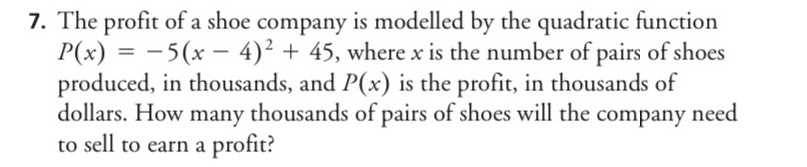### ¿Todavía tienes preguntas de matemáticas?

Pregunte a nuestros tutores expertos
Algebra
PreguntaThe profit of a shoe company is modelled by the quadratic function $$P ( x ) = - 5 ( x - 4 ) ^ { 2 } + 45 ,$$ where $$x$$ is the number of pairs of shoes produced, in thousands, and $$P ( x )$$ is the profit, in thousands of dollars. How many thousands of pairs of shoes will the company need to sell to earn a profit?

$$x> 1$$